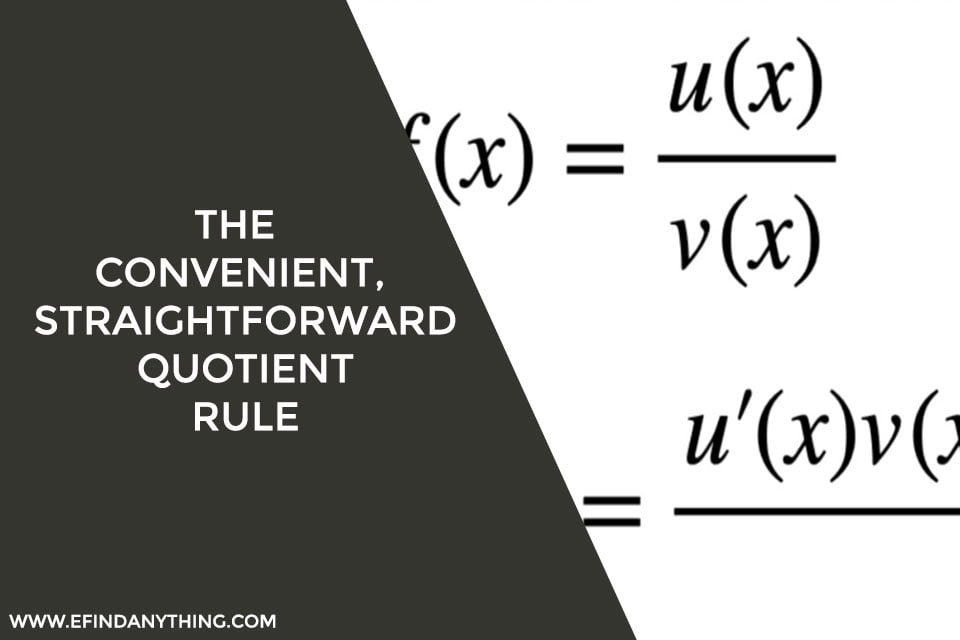## The Convenient, Straightforward Quotient Rule

In calculus, the quotient rule is a technique for determining the derivative of any function provided in the form of a quotient derived by dividing two differentiable functions. The quotient rule says that the derivative of a quotient is equal to the ratio of the result achieved by subtracting the numerator times the denominator’s derivative …